Select Page

### CAT 2022 Mensuration Practice Exercise-2

Geometry and Mensuration is one of the most important sections in Quantitative Analysis part of CAT. Many students have the wrong perception that geometry and mensuration problems are tough to crack. This is not completely true. With the help of these tests and examples you will be able to cruise through the section easily. Remember, the purpose of these CAT mensuration problems and solutions is to provide you relevant examples and practice material that helps you understand the subtle nuances for the topic.

Question 1: Three identical cones with base radius are placed on their bases so that each in touching the other two. The radius of the circle drawn through their vertices is:

(a) Smaller than
(b) Equal to
(c) Larger than
(d) Depends on the height of the cones

Answer: (c)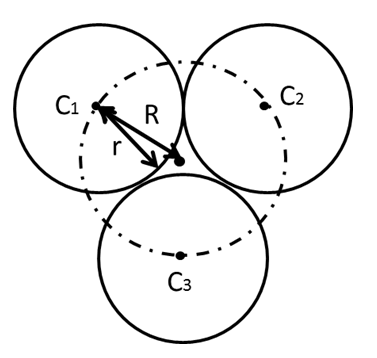The diagram drawn besides gives the picture of the base of the cones and a circle drawn from their centers. Let C1,C2 and C3 be the vertices of the three cones. r is the radius of these cones. Let R be the radius of the circle drawn through their centers. The diagram is drawn with estimation and clearly depicts that the radius of the circle drawn will be larger.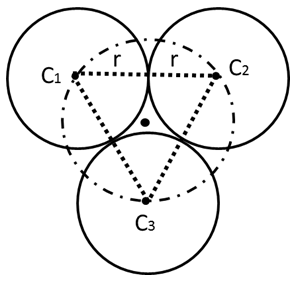Alternatively, the centers when joined form an equilateral triangle with side 2r. The circumcircle of this triangle will be our required circle. The circumradius of this circle will be

R = 2/√3 r = 1.155r > r Therefore, clearly R > r.

Question 2: A slab of ice 8 inches in length, 11 inches in breadth, and 2 inches thick was melted and re-solidified in the form of a rod of 8 inches diameter. The length of such a rod, in inches, in nearest to:

(a) 3
(b) 3·5
(c) 4
(d) 4·5

When the ice is melted and re-solidified the volume of water remains the same. Thus the volume of the slab of ice is equal to the volume of the rod. The slab of ice is in form of a cuboid whose volume is l×b×h, where length (l) is 8 inches, breadth (b) is 11 inches and height (h) is 2 inches

So, Volume of ice slab = l×b×h= 8× 11× 2 = 176 cubic inch

The rod is a type of cylinder. Volume is πr2h .

The diameter is 8 inches so the radius r  = 4 inches.

Hence volume of rod = volume of ice slab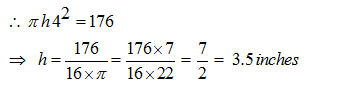Question 3: A right circular cone of height h is cut by a plane parallel to the base at a distance h/3 from the base, then the volumes of the resulting cone and the frustum are in the ratio :

(a) 1: 3
(b) 8: 19
(c) 1: 4
(d) 1: 7

The Plane cuts the cone at a height h/3 from the base as shown below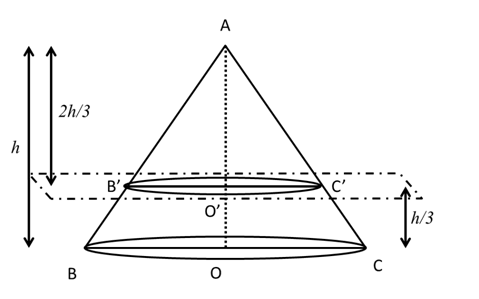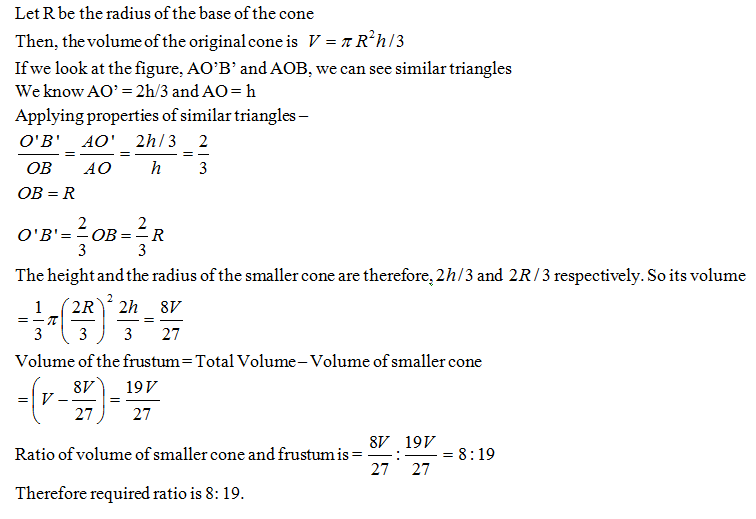Question 4: The length of a ladder is exactly equal to the height of the wall it is leaning against. The lower end of the ladder is kept on a stool of height 3 m and the stool is kept 9 m away from the wall, the upper end of the ladder coincides with the top of the wall. Then, the height of the wall is:

(a) 12 m
(b) 15 m
(c) 18 m
(d) 11 m

The ladder and the wall are of the same height

Then, Let the height of the wall be x m

Therefore height of the ladder will also be x m.

According to the question we can draw the following figure –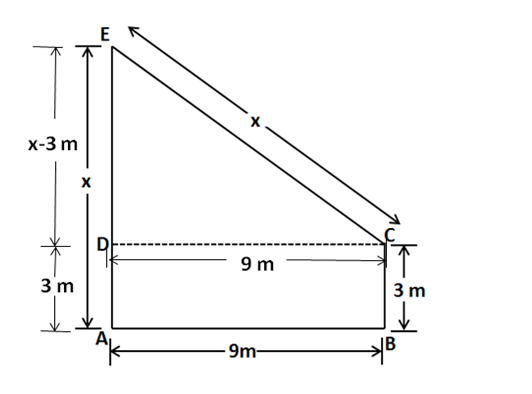DC = AB = 9m (distance of stool from the wall)

ED = EA – AD = (x – 3) m

In the Triangle EDC, Applying Pythagoras theorem as Wall stands at 90˚ to the floor,

ED2 +DC2 = EC2

(x -3)2 +92 = x2

x2 + 9 – 6x + 81=x2

6x=90

x=15

The height of the wall = x = 15 m

Question 5: In the adjoining figure, AC +AB = 5AD and AC – AD = 8. Then, the area of the rectangle ABCD is: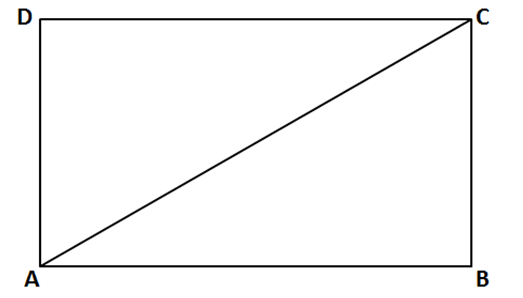(a) 36
(b) 50
(c) 60